Anúncio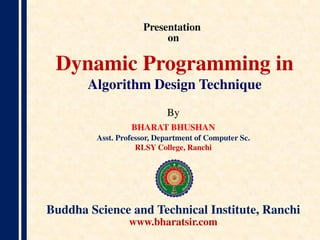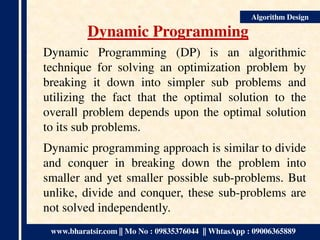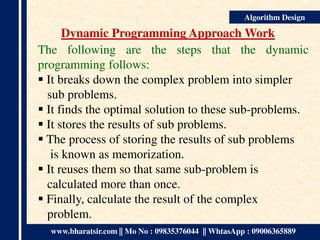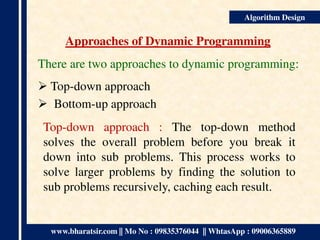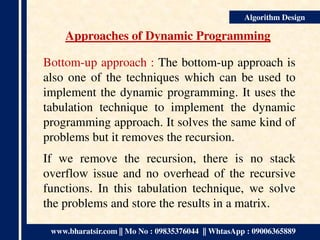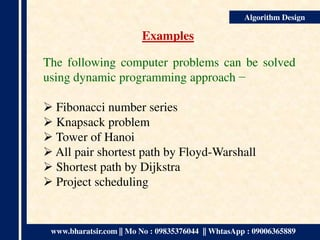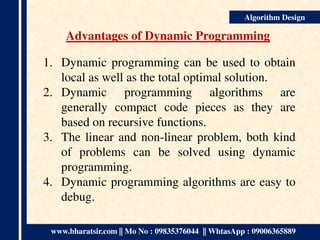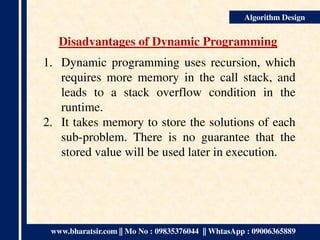1 de 9
Anúncio

### Dynamic Programming

1. Presentation on By BHARAT BHUSHAN Asst. Professor, Department of Computer Sc. RLSY College, Ranchi Buddha Science and Technical Institute, Ranchi www.bharatsir.com Dynamic Programming in Algorithm Design Technique
2. www.bharatsir.com || Mo No : 09835376044 || WhtasApp : 09006365889 Algorithm Design Dynamic Programming Dynamic Programming (DP) is an algorithmic technique for solving an optimization problem by breaking it down into simpler sub problems and utilizing the fact that the optimal solution to the overall problem depends upon the optimal solution to its sub problems. Dynamic programming approach is similar to divide and conquer in breaking down the problem into smaller and yet smaller possible sub-problems. But unlike, divide and conquer, these sub-problems are not solved independently.
3. www.bharatsir.com || Mo No : 09835376044 || WhtasApp : 09006365889 Algorithm Design Dynamic Programming Approach Work The following are the steps that the dynamic programming follows:  It breaks down the complex problem into simpler sub problems.  It finds the optimal solution to these sub-problems.  It stores the results of sub problems.  The process of storing the results of sub problems is known as memorization.  It reuses them so that same sub-problem is calculated more than once.  Finally, calculate the result of the complex problem.
4. www.bharatsir.com || Mo No : 09835376044 || WhtasApp : 09006365889 Algorithm Design Approaches of Dynamic Programming There are two approaches to dynamic programming:  Top-down approach  Bottom-up approach Top-down approach : The top-down method solves the overall problem before you break it down into sub problems. This process works to solve larger problems by finding the solution to sub problems recursively, caching each result.
5. www.bharatsir.com || Mo No : 09835376044 || WhtasApp : 09006365889 Algorithm Design Approaches of Dynamic Programming Bottom-up approach : The bottom-up approach is also one of the techniques which can be used to implement the dynamic programming. It uses the tabulation technique to implement the dynamic programming approach. It solves the same kind of problems but it removes the recursion. If we remove the recursion, there is no stack overflow issue and no overhead of the recursive functions. In this tabulation technique, we solve the problems and store the results in a matrix.
6. www.bharatsir.com || Mo No : 09835376044 || WhtasApp : 09006365889 Algorithm Design Examples The following computer problems can be solved using dynamic programming approach −  Fibonacci number series  Knapsack problem  Tower of Hanoi  All pair shortest path by Floyd-Warshall  Shortest path by Dijkstra  Project scheduling
7. www.bharatsir.com || Mo No : 09835376044 || WhtasApp : 09006365889 Algorithm Design Advantages of Dynamic Programming 1. Dynamic programming can be used to obtain local as well as the total optimal solution. 2. Dynamic programming algorithms are generally compact code pieces as they are based on recursive functions. 3. The linear and non-linear problem, both kind of problems can be solved using dynamic programming. 4. Dynamic programming algorithms are easy to debug.
8. www.bharatsir.com || Mo No : 09835376044 || WhtasApp : 09006365889 Algorithm Design Disadvantages of Dynamic Programming 1. Dynamic programming uses recursion, which requires more memory in the call stack, and leads to a stack overflow condition in the runtime. 2. It takes memory to store the solutions of each sub-problem. There is no guarantee that the stored value will be used later in execution.
9. Bharat Bhushan, Assistant Professor, RLSY College, Ranchi Algorithm Design
Anúncio